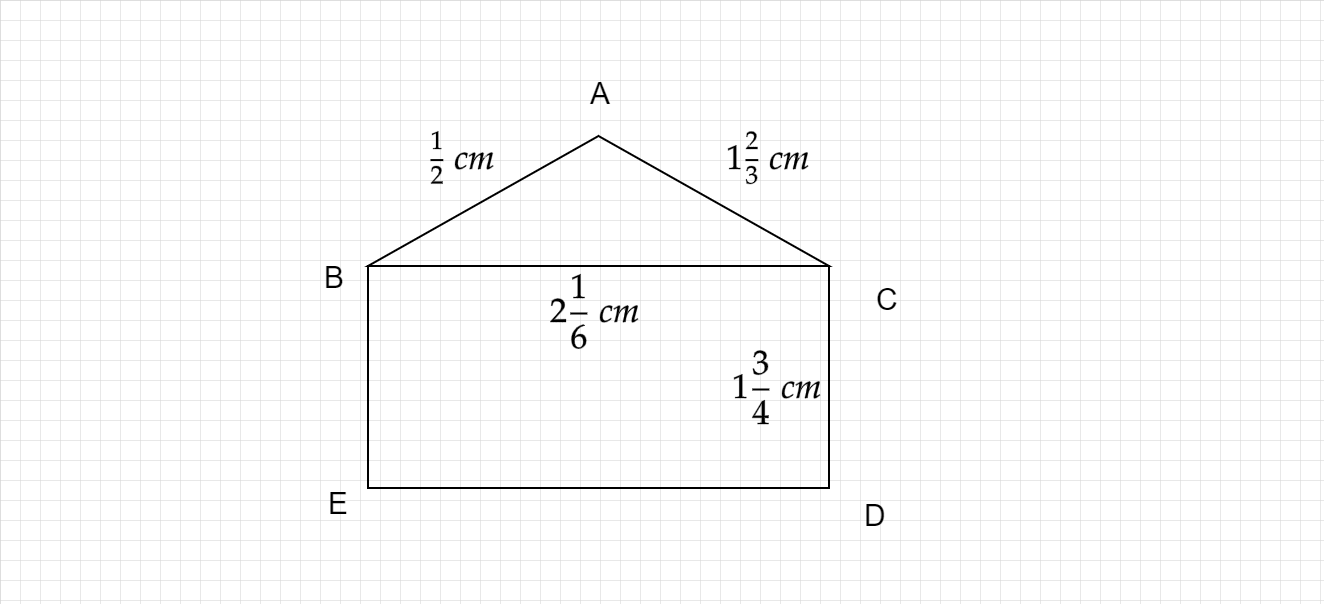"
">

# Find the Perimeter of Triangle ABC.Find the Perimeter of Rectangle BCDE. from the following figure."

Given :

ABCDE is the given figure.

To find :

We have to find the perimeters of Triangle ABC and Rectangle BCDE.

Solution :

Perimeter of a triangle of sides of lengths a units, b units and c units is $(a+b+c)$ units.

Perimeter of a rectangle of length l units and breadth b units is $2(l+b)$ units.

The lengths of the given sides of the triangle are $\frac{1}{2}$ cm, 1$\frac{2}{3}$ cm and 2$\frac{1}{6}$ cm.

The perimeter of the given triangle = $\left(\frac{1}{2} +1\frac{2}{3} +2\frac{1}{6}\right) \ cm\ =\left(\frac{1}{2} +\frac{5}{3} +\frac{13}{6}\right) cm=\left(\frac{1\times 6+5\times 2+13}{6}\right) cm=\frac{29}{6} cm=4\frac{5}{6} cm.\$

The lengths of the sides of the rectangle are 2$\frac{1}{6}$ cm and 1$\frac{3}{4}$ cm.

The perimeter of the given rectangle = $2\left( 2\frac{1}{6} +1\frac{3}{4}\right) \ cm\ =2\left(\frac{13}{6} +\frac{7}{4}\right) cm=2\left(\frac{13\times 2+7\times 3}{12}\right) cm=2\times \frac{47}{12} cm=\frac{47}{6} cm=7\frac{5}{6} cm.\$

Perimeter of Triangle ABC = $4\frac{5}{6}$ cm

Perimeter of Rectangle  BCDE = $7\frac{5}{6}$ cm

Updated on: 10-Oct-2022

41 Views